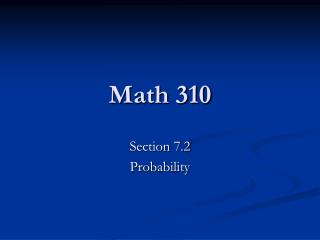Download PresentationMath 310

# Math 310 - PowerPoint PPT PresentationDownload Presentation## Math 310

- - - - - - - - - - - - - - - - - - - - - - - - - - - E N D - - - - - - - - - - - - - - - - - - - - - - - - - - -
##### Presentation Transcript

1. Math 310 Section 7.2 Probability

2. Succession of Events So far, our discussion of events have been in terms of a single stage scenario. We might be looking at the probability of more than one event occurring, however, we were only looking at that probability in the case of one “experiment.” Now we will look at the probability of a sequence of events occurring.

3. Ex. Looking at a single occurrence would be looking at one flip of a coin, or one role of a die. A succession of events would be rolling a die multiple times or flipping a coin multiple times. In a multistage scenario the sequence of stages represents a single event/outcome.

4. Tree Diagrams Tree diagrams are a way to represent a succession of events. Each branch represents one possible outcome.

5. H HH H T HT H TH T T TT Ex. Use a tree diagram to look at the possible outcomes of flipping a coin twice.

6. Probability along a branch Property The probability of an outcome along any path of a tree diagram is equal to the product of all the probabilities along the path.

7. H H HH = ¼ ½ T ½ ½ H HT = ¼ TH = ¼ ½ ½ T T ½ TT = ¼ Ex. Consider flipping a coin two times. What is the probability of getting a tail, and then another tail? What is the probability of getting a head and then a tail? What is the probability of getting at least one head?

8. Independent Events Two events are independent if the outcome of one event has no influence on the outcome of the second event.

9. Probability of Independent Events Property For any independent events E1 and E2, P(E1 ∩ E2) = P(E1)· P(E2)

10. NOTE Be careful of the notation used in the previous slide. It is reference to a succession of events. Multiple experiments if you will. Single Stage Experiment notation - P(A UB) Multi Stage Experiment notation - P(E1 ∩ E2)

11. Ex. Single Stage - Given a die what is the probability of rolling a die and getting a 1 or a 2. S = {1, 2, 3, 4, 5, 6}, A = {1}, B = {2} P(A UB) = P(A) + P(B) – P(A ∩ B) = 1/6 + 1/6 – 0, not P(A)· P(B) = 1/6 Multi Stage - Given a die what is the probability of rolling a die and getting a 1 and then a 2.

12. 1,3 = 1/36 3 1 ⅙ ⅙ 2 4 1,4 = 1/36 ⅙ ⅙ 3 ⅙ 4 Ex. Multi Stage - Given a die what is the probability of rolling a die and getting a 1 and then a 3.

13. Dependent Events In a succession of events, if two events are dependent, their outcomes influence each other. Note: One must take care in calculating the new probabilities along a branch in the tree diagram.

14. r r r r 6/17 6/17 6/17 6/16 p p p p 3/17 3/17 3/17 3/16 8/17 8/17 8/17 7/16 b b b b Ex. Suppose you have a bag with marbles in it: 8 blue, 6 red, 3 puce. What is the probability of drawing a blue, and then a red, without replacement? So the probability would be the product of the probabilities along the branch which yields: 48/272 or 3/17

15. Let us now do a series of examples further illustrating tree diagrams and the probability of a succession of events.

16. Ex. Take the word Mississippi. If each letter was on a piece of paper in a hat what is the probability of spelling sip by drawing out the letters in order with replacement? Without replacement? Looking along a branch of a tree diagram for this you would get: (4/11)· (4/11)· (2/11) with replacement (4/11)· (4/10)· (2/9) without replacement

17. Box 1 Box 2 Box 3 Ex. Consider the three boxes as shown: A marble is draw from box 1 and put into box 2. A marble is taken from box 3 and put into box 2. Then a marble is taken from box 2. What is the probability the marble is red? (2/3)· 1· (1/2) + (1/3)· 1· (1/4) = 1/3

18. Ex. (pg 456) “Consider the following game, which Arthur and Gwen play: There are two colored marbles and one white marble in a box. Gwen mixes the marbles, and Arthur draws two marbles at random without replacement. If the two marbles match, Arthur wins; otherwise Gwen wins. Does each player have an equal chance of winning?’

19. Ex. How would you make the previous game fair?